In :
import numpy as np
import scipy as sp
import scipy.stats as stats
import codecs
import nltk
import lda
import sklearn
import string
import cPickle as pickle
import matplotlib.pyplot as plt
import collections, operator
import pandas as pd
import seaborn as sns
import matplotlib.gridspec as gridspec
import numpy.matlib
from matplotlib import animation
from scipy.special import gammaln
from nltk.corpus import stopwords
from nltk.stem.porter import *
from collections import Counter, defaultdict
from sklearn.preprocessing import normalize
from sklearn.feature_extraction.text import TfidfTransformer, CountVectorizer
from collections import defaultdict
from mpl_toolkits.mplot3d.axes3d import Axes3D
from matplotlib.ticker import LinearLocator, FormatStrFormatter
from wordcloud import WordCloud
plt.style.use("ggplot"); plt.style.use("bmh");
%matplotlib inline


# 1. Introduction¶

LDA is a generative probabilistic model for collections of grouped discrete data. Each group is described as a random mixture over a set of latent topics where each topic is a discrete distribution over the collection’s vocabulary. We use Gibbs sampling to sample from the posterior of the distribution described by LDA to extract thematic content from ten classic novels. We train on half of the pages, and perform inference on the remainder. We use nearest neighbor on the queried topic distibution to query the closest match. We were able to correctly label 100% of our test data with the correct title.

# 2. Methodology¶

## 2.1. Pre-processing¶

• Our first step is to load the data from a folder containing all ten of the classic novels which compose our training corpus
In :
import codecs
books = ["beowulf.txt", "divine_comedy.txt", "dracula.txt", "frankenstein.txt", "huck_finn.txt", "moby_dick.txt", "sherlock_holmes.txt", "tale_of_two_cities.txt", "the_republic.txt", "ulysses.txt"]
all_docs = []
for book in books:
with codecs.open('data/%s'%(book), 'r', encoding='utf-8') as f:
all_docs.append(" ".join(lines))

• We remove punctuation and numbers from our books.
• Additionally, we remove stop words, or words that don't have much lexical meaning, ie: "the, is, at, which, on...".
In :
stemmer = PorterStemmer()

# def remove_insignificant_words(processed_docs, min_thresh = 9, intra_doc_thresh = .9):
#     all_tokens = np.unique([item for sublist in processed_docs for item in sublist])
#     low_freq_words = [k for k, v in Counter(all_tokens).iteritems() if v < min_thresh]
#     high_freq_words = []
#     for word in all_tokens:
#         num_docs_containing_word = np.sum(map(lambda doc: word in doc, processed_docs))
#         if float(num_docs_containing_word) / len(processed_docs) >= intra_doc_thresh:
#             high_freq_words.append(word)
#     words_to_remove = set(low_freq_words + high_freq_words)
#     return map(lambda doc_tokens: [w for w in doc_tokens if w not in words_to_remove], processed_docs)

def stem_tokens(tokens, stemmer):
stemmed = []
for item in tokens:
stemmed.append(stemmer.stem(item))
return stemmed

def tokenize_and_remove_grammar_numbers_stopwords(doc):
doc = doc.lower()
no_punctuation = re.sub(r'[^a-zA-Z\s]','',doc)
tokens = nltk.word_tokenize(no_punctuation)
filtered = [w for w in tokens if not w in stopwords.words('english')]
#stemmed = stem_tokens(filtered, stemmer)
#return stemmed
return filtered

processed_docs = np.array(map(tokenize_and_remove_grammar_numbers_stopwords, all_docs))
#processed_docs = remove_insignificant_words(processed_docs, all_tokens)

In :
processed_docs[500:510]

Out:
[u'tread',
u'warrior',
u'mail',
u'viii',
u'english',
u'translations',
u'beowulf',
u'professor',
u'garnett',
u'alone']
In :
np.save("temp_data/processed_docs.npy", processed_docs)

In :
processed_docs = np.load("temp_data/processed_docs.npy")


## 2.2 Build vocabulary¶

In :
vocab = np.unique(np.hstack(processed_docs.flat))
vocab_dict = {}
inv_vocab_dict = {}
for idx, w in enumerate(vocab):
vocab_dict[w] = idx
inv_vocab_dict[idx] = w

In :
vocab[np.random.choice(vocab.size, 10)]

Out:
array([u'tarpeian', u'capstans', u'pairs', u'fetid', u'na',
dtype='<U69')

## 2.3 Map Docs to Vocab¶

• We now translate our documents into the language of numbers, allowing us to perform operations on our data
In :
docs_as_nums = map(lambda doc: [vocab_dict[w] for w in doc], processed_docs)
docs_as_nums[:10]

Out:
[39038, 22075, 15318, 4485, 15318, 53645, 1932, 1940, 10849, 1375]

## 2.4 Remove Low Frequency Words and Words that Appear Across >= 90% of Documents¶

• We remove words that will contribute very little to the signal we use to distinguish documents
In :
def freq_map(doc):
out = np.zeros(vocab.size, dtype=np.int32)
for w in doc:
out[w] += 1
return out

In :
count_mat =np.array(map(freq_map, np.array(docs_as_nums)), dtype=np.int32)
low_freq_words = np.where(np.sum(count_mat != 0, axis=0) < 2)
high_freq_words = np.where(np.sum(count_mat > 0, axis=0) >= .9*count_mat.shape)
words_to_remove = np.unique(np.append(low_freq_words, high_freq_words))

In :
docs_as_nums = map(lambda doc: [word for word in doc if word not in words_to_remove], docs_as_nums)

In :
count_mat =np.array(map(freq_map, np.array(docs_as_nums)), dtype=np.int32)

In :
np.save("temp_data/docs_as_nums.npy", np.array(docs_as_nums))

In :
docs_as_nums = np.load("temp_data/docs_as_nums.npy")


## 2.5 Build Training and Test Set¶

• We split each of the books in half to use a training data and as test data, respectively.
In :
test_docs, train_docs = [], []
for doc in docs_as_nums:
test_docs.append(np.array(doc[0:len(doc)/2]))
train_docs.append(np.array(doc[len(doc)/2:]))
test_docs, train_docs = np.array(test_docs), np.array(train_docs)

In :
test_docs

Out:
array([array([56863,  1728, 16395, ..., 53486, 29188,  3112]),
array([22076, 14172,  9563, ..., 50365, 13691, 50589]),
array([ 3030,  8565, 39104, ...,  2742, 31975, 33093]),
array([22076, 30986, 44646, ..., 17849, 19401, 16044]),
array([  649, 18404, 52126, ..., 37781, 32764, 28716]),
array([13364, 55565, 56864, ..., 21574, 43539, 41253]),
array([22076,   649, 44675, ..., 11543, 36011, 16842]),
array([ 8731,  8138, 13368, ..., 27002, 39068, 32912]),
array([41306, 37635, 56864, ..., 36256, 13035, 52297]),
array([52350, 27031, 56864, ...,  9509, 15737, 19274])], dtype=object)

## 2.6 Build a Count Matrix¶

A count matrix is built by setting each row equal to the number of times a vocabulary word is used in a document. The count matrix has dimensions (num_docs x size_of_vocab). We need the count matrix because our LDA function will take it as an input.

In :
train_count_mat = np.array(map(freq_map, train_docs), dtype=np.int32)
test_count_mat = np.array(map(freq_map, test_docs), dtype=np.int32)

In :
train_count_mat

Out:
array([[0, 0, 0, ..., 0, 0, 0],
[0, 0, 0, ..., 0, 0, 0],
[0, 0, 0, ..., 0, 0, 0],
...,
[0, 0, 0, ..., 0, 0, 0],
[0, 0, 0, ..., 0, 0, 0],
[0, 0, 0, ..., 0, 0, 0]], dtype=int32)
In :
np.save("temp_data/train_count_mat.npy", train_count_mat)
np.save("temp_data/test_count_mat.npy", test_count_mat)

In :
train_count_mat = np.load("temp_data/train_count_mat.npy")


## 3. LDA with Gibbs Sampling¶

LDA is a generative probabilistic model for collections of grouped discrete data. Each group is described as a random mixture over a set of latent topics where each topic is a discrete distribution over the collection’s vocabulary. Algorithm 1 delineates how we can draw from the posterior of the LDA model using Gibbs Sampling

We define the following parameters whose relationship is described by the plate notation in Figure 1.

• α is the parameter of the Dirichlet prior on the per-document topic distributions,
• β is the parameter of the Dirichlet prior on the per-topic word distribution,
• $\theta_i$ is the topic distribution for document i,
• $\phi_k$ is the word distribution for topic k,
• $z_{ij}$ is the topic for the jth word in document i, and
• $w_{ij}$ is the specific word.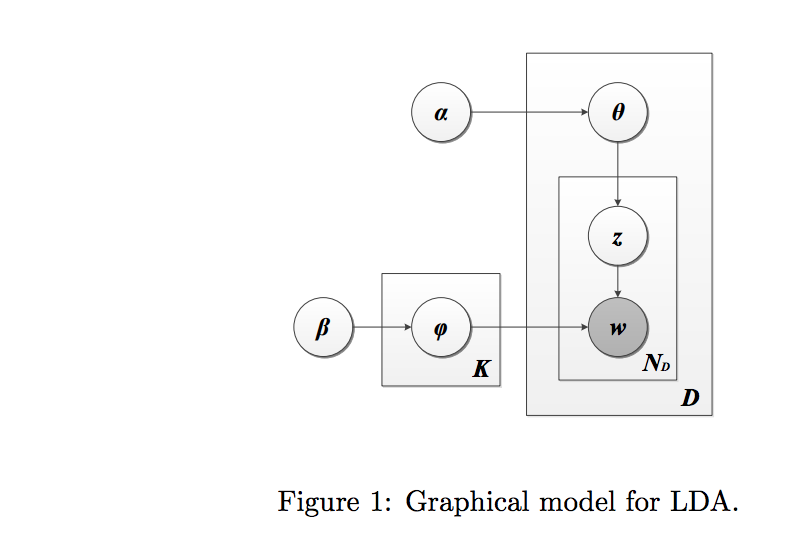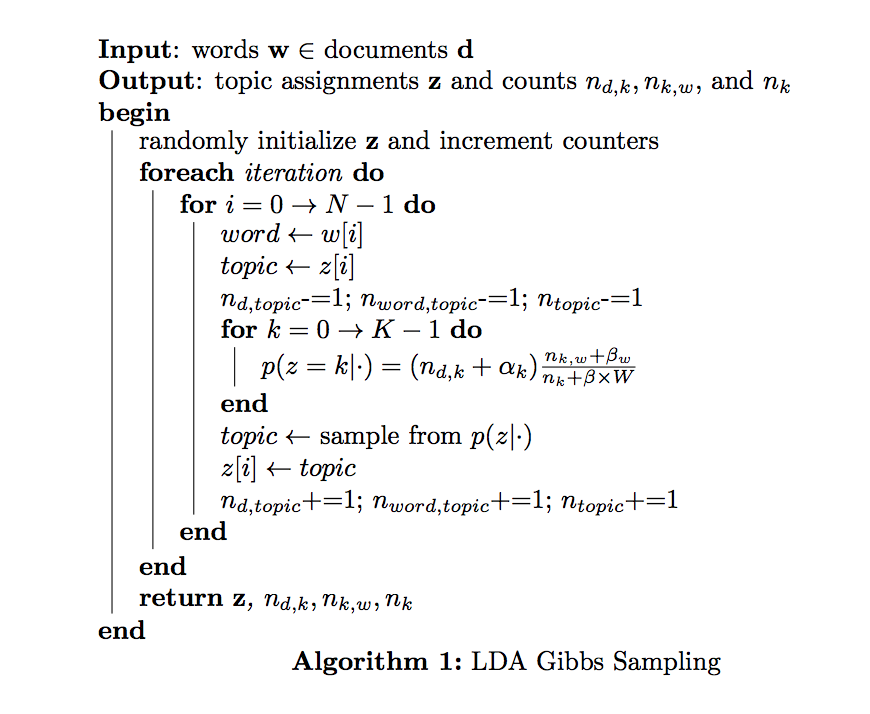• First, let's define our conditional distribution
In :
def conditional_dist(alpha, beta, nwt, nd, nt, d, w):
"""
Compute the conditional distribution
"""
W = nwt.shape
p_z =  (ndt[d,:] + alpha) * ((nwt[w,:] + beta) / (nt + beta * W))
# normalization
p_z /= np.sum(p_z)
return p_z

• We'll also need the log likelihood to verify that our model is converging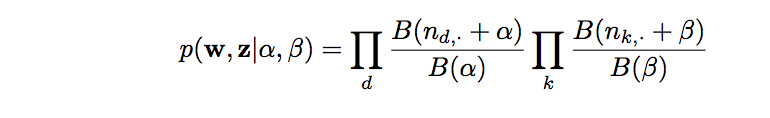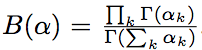In :
def log_likelihood(alpha, beta, nwt, ndt, n_topics):
"""
Compute the likelihood that the model generated the data.
"""
W = nwt.shape
n_docs = ndt.shape
likelihood = 0

for t in xrange(n_topics):
likelihood += log_multinomial_beta(nwt[:,t]+beta) - log_multinomial_beta(beta, W)

for d in xrange(n_docs):
likelihood += log_multinomial_beta(ndt[d,:]+alpha) - log_multinomial_beta(alpha, n_topics)

return likelihood

def log_multinomial_beta(alpha, K=None):
"""
Logarithm of the multinomial beta function.
"""
if K is None:
return np.sum(gammaln(alpha)) - gammaln(np.sum(alpha))
else:
return K * gammaln(alpha) - gammaln(K*alpha)

• Since our input is a count matrix, we need to recover our document by multiplying the token by its frequency and combining (in any order since we have a bag of words assumption)
In :
def word_indices(arr):
"""
Transform a row of the count matrix into a document by replicating the token by its frequency
"""
for idx in arr.nonzero():
for i in xrange(int(arr[idx])):
yield idx

• To perform LDA with Gibbs Sampling we need to initialize z randomly and initialize our counters.
• We set the number of topics to 1000.
In :
n_topics = 15
alpha = .1 # prior weight of topic k in a document; few topics per document
beta = 0.05 # prior weight of word w in a topic; few words per topic
n_docs, W = train_count_mat.shape
# number of times document m and topic z co-occur
ndt = np.zeros((n_docs, n_topics))
# number of times word w and topic z co-occur
nwt = np.zeros((W, n_topics))
nd = np.zeros(n_docs)
nt = np.zeros(n_topics)
iters = 25
topics = defaultdict(dict)
delta_topics = []
delta_doc_topics = defaultdict(list)
likelihoods = []

for d in xrange(n_docs):
# i is a number between 0 and doc_length-1
# w is a number between 0 and W-1
for i, w in enumerate(word_indices(train_count_mat[d, :])):
# choose an arbitrary topic as first topic for word i
t = np.random.randint(n_topics)
ndt[d,t] += 1
nd[d] += 1
nwt[w,t] += 1
nt[t] += 1
topics[d][i] = t

• Now, we do Gibbs sampling for 25 iterations
In :
# for each iteration
for it in xrange(iters):
delta_topics_iteration = 0
# for each doc
for d in xrange(n_docs):
delta_doc_topics_iteration = 0
# for each word
for i, w in enumerate(word_indices(train_count_mat[d, :])):
# get topic of mth document, ith word
t = topics[d][i]
# decrement counters
ndt[d,t] -= 1; nd[d] -= 1; nwt[w,t] -= 1; nt[t] -= 1

p_z = conditional_dist(alpha, beta, nwt, nd, nt, d, w)
t = np.random.multinomial(1,p_z).argmax()

# increment counters
ndt[d,t] += 1; nd[d] += 1; nwt[w,t] += 1; nt[t] += 1;
# increment convergence counter if the value for topic changes
if topics[d][i] != t:
delta_doc_topics_iteration += 1
delta_topics_iteration += 1

topics[d][i] = t

delta_doc_topics[d].append(delta_doc_topics_iteration)

print "-"*50, "\n Iteration", it+1, "\n", "-"*50, "\n"
likelihood = log_likelihood(alpha, beta, nwt, ndt, n_topics)
print "Likelihood", likelihood
likelihoods.append(likelihood)
print "Delta topics", delta_topics_iteration, "\n"
delta_topics.append(delta_topics_iteration)


--------------------------------------------------
Iteration 1
--------------------------------------------------

Likelihood -1509564.20778
Delta topics 111185

--------------------------------------------------
Iteration 2
--------------------------------------------------

Likelihood -1460465.93552
Delta topics 92721

--------------------------------------------------
Iteration 3
--------------------------------------------------

Likelihood -1429010.49736
Delta topics 82885

--------------------------------------------------
Iteration 4
--------------------------------------------------

Likelihood -1399769.43548
Delta topics 75405

--------------------------------------------------
Iteration 5
--------------------------------------------------

Likelihood -1374871.8865
Delta topics 68348

--------------------------------------------------
Iteration 6
--------------------------------------------------

Likelihood -1354150.78614
Delta topics 62542

--------------------------------------------------
Iteration 7
--------------------------------------------------

Likelihood -1336644.63968
Delta topics 57418

--------------------------------------------------
Iteration 8
--------------------------------------------------

Likelihood -1322776.15127
Delta topics 53710

--------------------------------------------------
Iteration 9
--------------------------------------------------

Likelihood -1312190.89999
Delta topics 50808

--------------------------------------------------
Iteration 10
--------------------------------------------------

Likelihood -1302767.39681
Delta topics 47879

--------------------------------------------------
Iteration 11
--------------------------------------------------

Likelihood -1295224.87184
Delta topics 46221

--------------------------------------------------
Iteration 12
--------------------------------------------------

Likelihood -1290369.05947
Delta topics 44605

--------------------------------------------------
Iteration 13
--------------------------------------------------

Likelihood -1285797.8575
Delta topics 43785

--------------------------------------------------
Iteration 14
--------------------------------------------------

Likelihood -1282721.62032
Delta topics 43039

--------------------------------------------------
Iteration 15
--------------------------------------------------

Likelihood -1278667.0523
Delta topics 42009

--------------------------------------------------
Iteration 16
--------------------------------------------------

Likelihood -1275429.60741
Delta topics 40992

--------------------------------------------------
Iteration 17
--------------------------------------------------

Likelihood -1273389.60434
Delta topics 40604

--------------------------------------------------
Iteration 18
--------------------------------------------------

Likelihood -1270359.51444
Delta topics 40144

--------------------------------------------------
Iteration 19
--------------------------------------------------

Likelihood -1268859.81599
Delta topics 39459

--------------------------------------------------
Iteration 20
--------------------------------------------------

Likelihood -1266553.22391
Delta topics 39338

--------------------------------------------------
Iteration 21
--------------------------------------------------

Likelihood -1264753.92816
Delta topics 38710

--------------------------------------------------
Iteration 22
--------------------------------------------------

Likelihood -1262537.89211
Delta topics 38319

--------------------------------------------------
Iteration 23
--------------------------------------------------

Likelihood -1260408.32057
Delta topics 37928

--------------------------------------------------
Iteration 24
--------------------------------------------------

Likelihood -1258189.39382
Delta topics 37435

--------------------------------------------------
Iteration 25
--------------------------------------------------

Likelihood -1256894.63423
Delta topics 36876



# 4. Analysis¶

### 4.1 Log Likelihood¶

We verify that the likelihood that our model generated the data increases over ever iteration. For convergence, we want to see a plateau, such that we are seeing diminishing gains in our log likelihood. As the graph below illustrates, this is exactly the case.

In :
plt.style.use("ggplot");plt.style.use("bmh");

ax = plt.figure(figsize=(14,4))
plt.plot(np.arange(25), likelihoods)
plt.title("Log Likelihood vs Iterations", fontsize="xx-large")
plt.xlabel("Iteration", fontsize="xx-large")
plt.ylabel("Log Likelihood", fontsize="xx-large")
plt.show()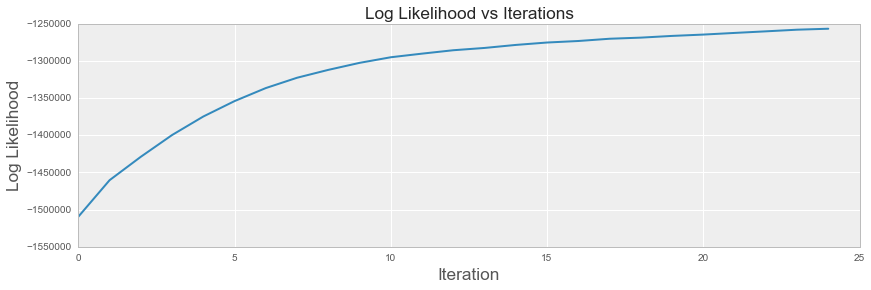### 4.2 Aggregate Word-Topic Assignment Swaps¶

We present a custom statistic to measure the total number of words whose topic assignment changed between iterations. We know that if the algorithm converges, the number of swaps every iteration should level out. The graph below illustrates this trend.

In :
plt.figure(figsize=(14,4))
plt.plot(np.arange(iters), delta_topics)
plt.title("Aggregate Swaps in Topic Assignments vs Iterations", fontsize="xx-large")
plt.xlabel("Iteration", fontsize="xx-large")
plt.ylabel("Aggregate Swaps in Topic Assignments", fontsize="xx-large")
plt.show()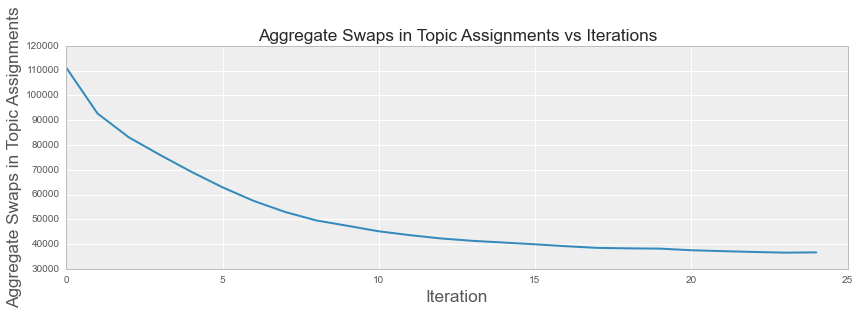### 4.3 Aggregate Word-Topic Assignment Swaps per Document¶

We apply the word-topic assignment swaps to a per-document basis. We should still see that on a document granularity, word-topic assignments should plateau. Each of the ten documents below illustrate this trend

In :
plt.figure(figsize=(16,25))
gs = gridspec.GridSpec(5, 2)

for i in range(len(books)):
ax = plt.subplot(gs[i])
ax.plot(np.arange(iters), delta_doc_topics[i])
ax.set_title("Aggregate Swaps in Topic Assignments vs Iterations for %s" %(books[i]), fontsize="medium")
ax.set_xlabel("Iteration", fontsize="medium")
ax.set_ylabel("Aggregate Swaps in Topic Assignments", fontsize="medium")

plt.show()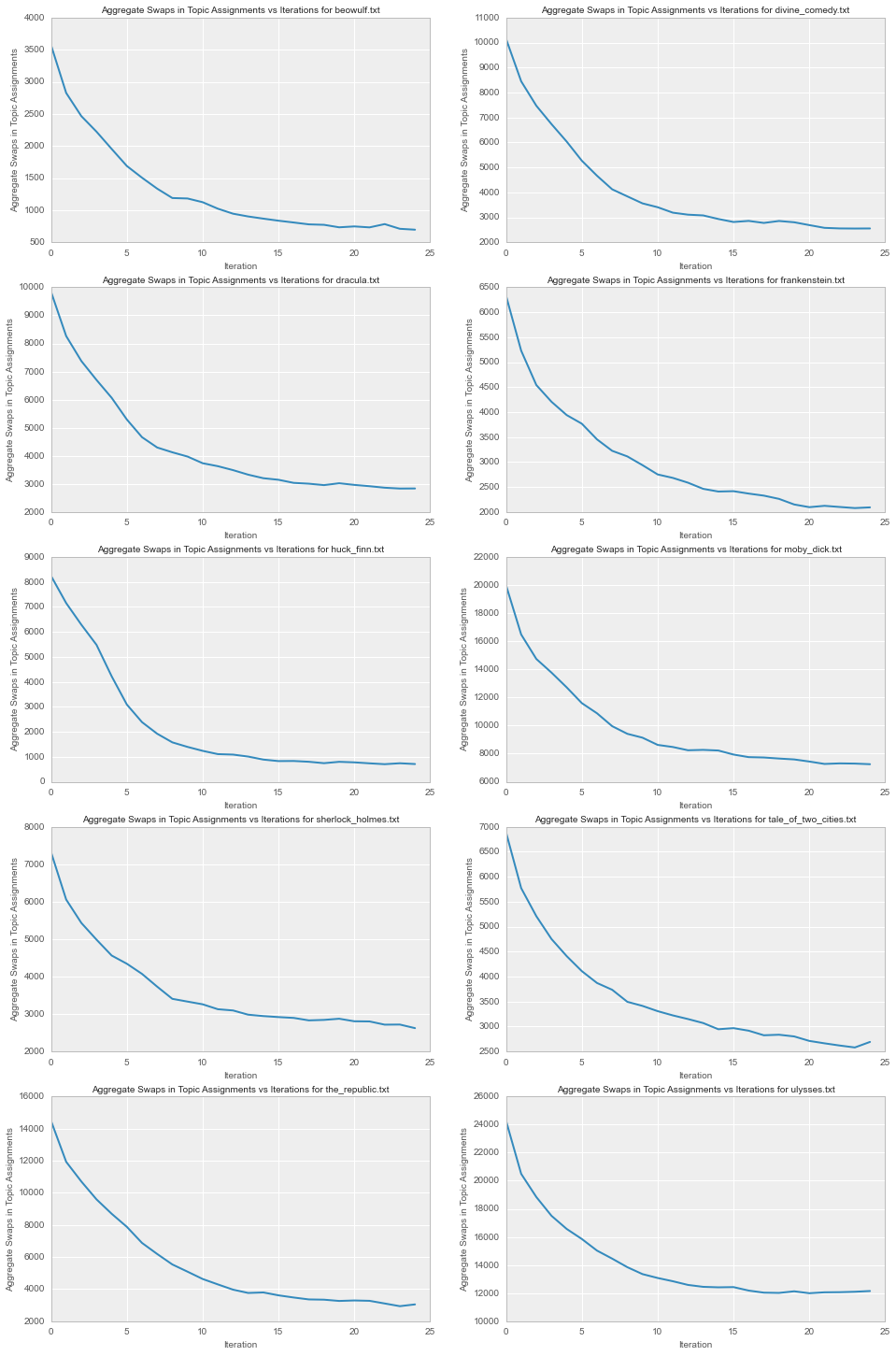### 4.4 Autocorrelation of Swaps¶

In :
plt.acorr(delta_topics-np.mean(delta_topics), ls='-', normed=True, usevlines=False, maxlags=iters-5, label=u'Shuffled')
plt.xlim([0,iters-5])
plt.title("Autocorrelation of Swaps")
plt.xlabel("Lags")
plt.ylabel("Autocorrelation")
plt.show()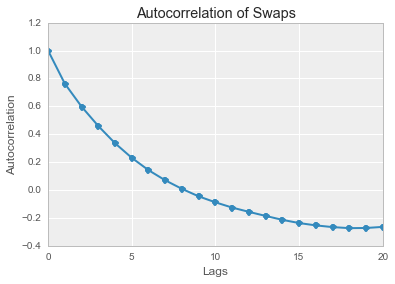### 4.5 Topics as a Distribution over Words¶

• One important output of LDA is a matrix of topics where each topic is a distribution over the vocabulary.
• We want to verify that we observe only a few high-mass words per topic since we set our beta parameter to a small number (.5)
In :
topic_words = defaultdict(lambda: [])
for d in xrange(n_docs):
for i, w in enumerate(word_indices(train_count_mat[d, :])):
t = topics[d][i]
topic_words[t].append(inv_vocab_dict[w])

# Normalize
for topic in topic_words.keys():
norm_topic_words = Counter(topic_words[topic])
total = sum(norm_topic_words.values(), 0.0)
for key in norm_topic_words:
norm_topic_words[key] /= total
topic_words[topic] = norm_topic_words

• Let's see what sort of topics LDA discovered. We will choose two topics at random
In :
for i in np.random.choice(n_topics, 2):
if topic_words[i]:
sorted_topic_words = sorted(topic_words[i].items(), key=operator.itemgetter(1), reverse=True)
print "\nMost important words for topic", i
for word in sorted_topic_words[:10]:
print word, word

Most important words for topic 0
thou 0.0776546939689
thy 0.0375965088956
thee 0.0286449591586
hath 0.0129797471187
spake 0.010518070941
een 0.00805639476334
cried 0.00794450039163
beheld 0.00749692290478
lo 0.00570661295737
doth 0.00559471858566

Most important words for topic 2
holmes 0.0204101280447
mr 0.0155964186002
really 0.0118417252335
proceed 0.00616154808896
influence 0.00548762876673
window 0.00539135457784
character 0.00529508038895
probably 0.00510253201117
observe 0.0048137094445
danger 0.0048137094445

• We can also visualize these topics as wordclouds
In :
plt.figure(figsize=(17,10))
gs = gridspec.GridSpec(1, 2)

ax = plt.subplot(gs)
wc = WordCloud(font_path="Verdana.ttf", background_color="white")
wc.generate(" ".join([ (" " + word)*int(1000*word) for word in topic_words.items()]))
ax.imshow(wc)
plt.axis("off")
ax.set_title("Word cloud for Topic 1\n")

ax = plt.subplot(gs)
wc = WordCloud(font_path="Verdana.ttf", background_color="white")
wc.generate(" ".join([ (" " + word)*int(1000*word) for word in topic_words.items()]))
plt.imshow(wc)
plt.axis("off")
ax.set_title("Word cloud for Topic 3\n")

plt.show()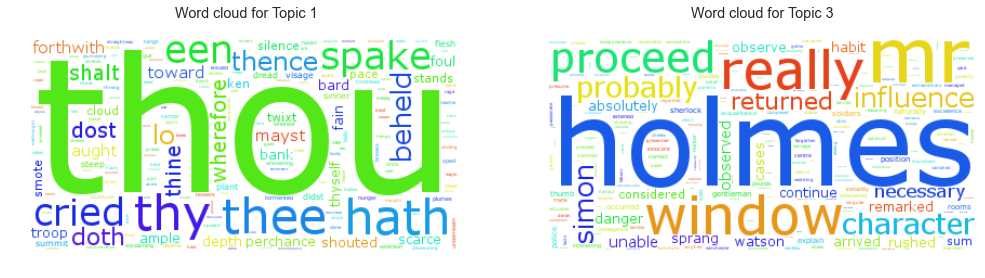• Because we set our parameters to ensure sparsity over topics, each topic should be only described by a few words. Let's see a histogram to verify that the sparsity constraint was realized.
In :
num_words_per_topic = [len(words) for topic, words in topic_words.iteritems()]
plt.figure(figsize=(14,5))
plt.hist(num_words_per_topic, bins=18, normed=True, histtype='stepfilled')
plt.title("Distribution of Number of Words per topic", fontsize="xx-large")
plt.xlabel("Normalized of Words")
plt.ylabel("Number of Topics")
plt.show()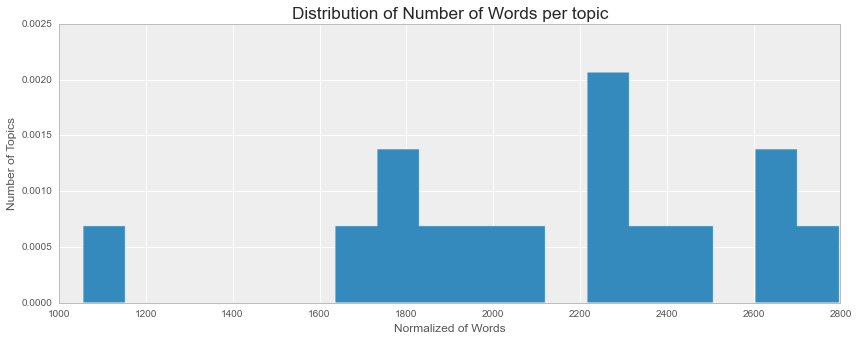### 4.6 Documents as a Distribution over Topics¶

• Let's find our topic distributions over the train documents.
• We want to verify that we observe few high-mass topics per document since we set our alpha parameter to a large number (.8)
In :
train_doc_topic_dist = np.zeros((n_docs, n_topics))
for d in xrange(n_docs):
# for each word
for i, w in enumerate(word_indices(train_count_mat[d, :])):
# get topic of mth document, ith word
z = topics[d][i]
train_doc_topic_dist[d, z] += 1

# NORMALIZE TOPIC DISTRIBUTION
row_sums = train_doc_topic_dist.sum(axis=1)
train_doc_topic_dist = train_doc_topic_dist / row_sums[:, np.newaxis]

In :
doc_topic_dist_df = pd.DataFrame(train_doc_topic_dist, columns=(["Topic " + str(i) for i in range(n_topics)]), index=([books[i] for i in range(n_docs)]))
doc_topic_dist_df

Out:
Topic 0 Topic 1 Topic 2 Topic 3 Topic 4 Topic 5 Topic 6 Topic 7 Topic 8 Topic 9 Topic 10 Topic 11 Topic 12 Topic 13 Topic 14
beowulf.txt 0.043277 0.006698 0.014168 0.000000 0.037609 0.000000 0.012880 0.000000 0.000000 0.000515 0.094024 0.785935 0.000000 0.002061 0.002834
divine_comedy.txt 0.611672 0.006585 0.003518 0.003428 0.000090 0.002887 0.080281 0.061068 0.009020 0.003608 0.021469 0.196284 0.000090 0.000000 0.000000
dracula.txt 0.007344 0.012736 0.072790 0.002231 0.036720 0.060426 0.035419 0.048805 0.023241 0.630008 0.016640 0.004090 0.015246 0.001301 0.033002
frankenstein.txt 0.025014 0.010435 0.112779 0.004145 0.207261 0.002430 0.441109 0.076329 0.001858 0.070469 0.033591 0.013150 0.001429 0.000000 0.000000
huck_finn.txt 0.000000 0.002877 0.001992 0.005755 0.000000 0.000664 0.000000 0.003541 0.002877 0.013834 0.000111 0.000111 0.000000 0.113324 0.854914
moby_dick.txt 0.063299 0.462426 0.031093 0.031360 0.026905 0.015457 0.020892 0.196356 0.023253 0.018709 0.030603 0.024901 0.022184 0.002539 0.030024
sherlock_holmes.txt 0.000000 0.002899 0.474396 0.028140 0.082367 0.070652 0.012923 0.057729 0.026570 0.149758 0.004348 0.000000 0.062077 0.000121 0.028019
tale_of_two_cities.txt 0.013136 0.007200 0.123153 0.492611 0.027536 0.015157 0.069850 0.046609 0.041935 0.100796 0.017557 0.005179 0.021346 0.000884 0.017052
the_republic.txt 0.007595 0.000060 0.103382 0.001145 0.044728 0.000000 0.247694 0.001989 0.000000 0.003617 0.579963 0.005847 0.002954 0.000784 0.000241
ulysses.txt 0.023397 0.028860 0.049234 0.025424 0.023363 0.132344 0.031883 0.045970 0.119151 0.030990 0.040198 0.016629 0.331684 0.044664 0.056208

First, we can look at a heatmap of our topics over documents

In :
plt.figure(figsize=(16,10))
sns.heatmap(doc_topic_dist_df)
plt.gca().axes.get_xaxis().set_ticks([])
plt.xticks(np.arange(n_topics)+.5, ["Topic " + str(i+1) for i in range(n_topics)], rotation="vertical")
plt.title("Heatmap of Topic Mass Across Documents\n", fontsize="xx-large")
plt.show()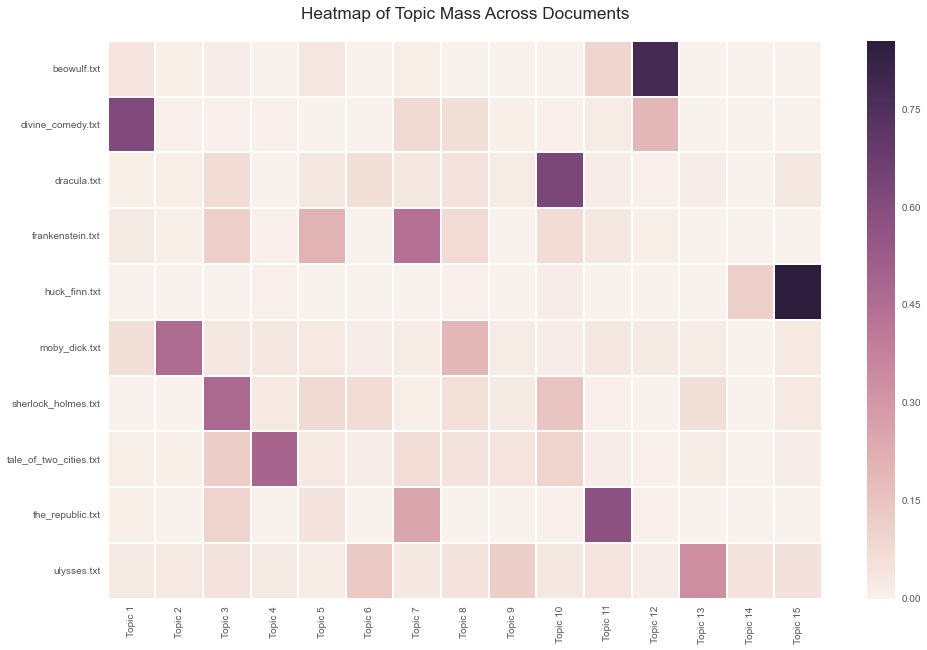In :
doc_topic_dist_df.plot(kind='bar', figsize=(16,5), stacked="true", title="Distribution of Topics per Document\n", fontsize="xx-large");
plt.legend(bbox_to_anchor=(1.1,1.05))
plt.show()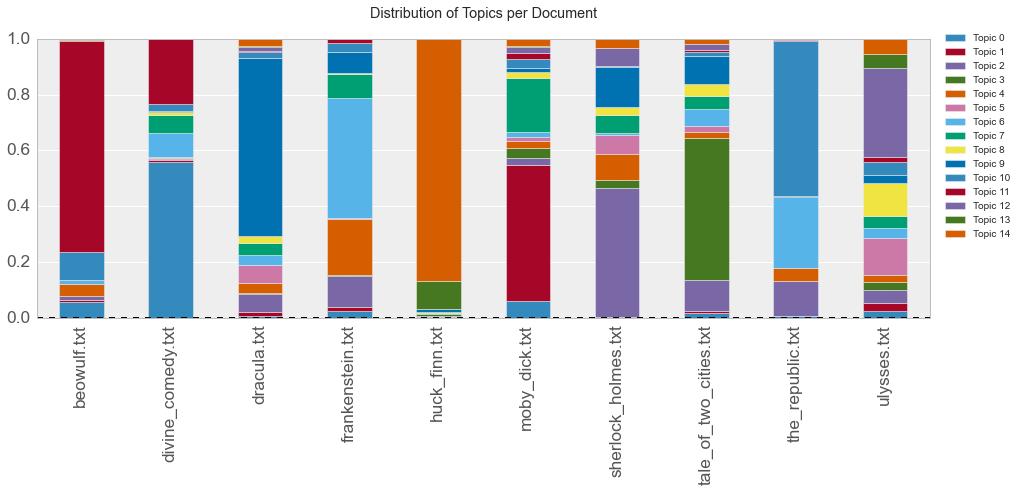### 4.7 Topic Landscape of Documents¶

In :
# Create an init function and the animate functions.
# Both are explained in the tutorial. Since we are changing
# the the elevation and azimuth and no objects are really
# changed on the plot we don't have to return anything from
# the init and animate function. (return value is explained
# in the tutorial.
def init():
# Create a figure and a 3D Axes
xx,yy = np.meshgrid(np.arange(n_topics),np.arange(n_docs)) # Define a mesh grid in the region of interest
zz=train_doc_topic_dist
surf = ax.plot_surface(xx, yy, zz, rstride=1, cstride=1, cmap=plt.cm.coolwarm, linewidth=0, antialiased=False)
ax.view_init(elev=50., azim=250)
ax.set_zlim(0.0001, np.max(train_doc_topic_dist)*1.1)
ax.zaxis.set_major_locator(LinearLocator(10))
ax.zaxis.set_major_formatter(FormatStrFormatter('%.02f'))
ax.set_yticklabels(books, rotation='vertical')
ax.set_xlabel("Topics")
ax.set_ylabel("")
fig.colorbar(surf, shrink=0.5, aspect=5)

def animate(i):
ax.view_init(elev=5., azim=i)

# Animate
fig = plt.figure(figsize=(10,5))
ax = Axes3D(fig)
anim = animation.FuncAnimation(fig, animate, init_func=init,
frames=360, interval=20, blit=True)
# Save
anim.save('ipynb_assets/topic_dist_3D.mp4', fps=30, extra_args=['-vcodec', 'libx264'])